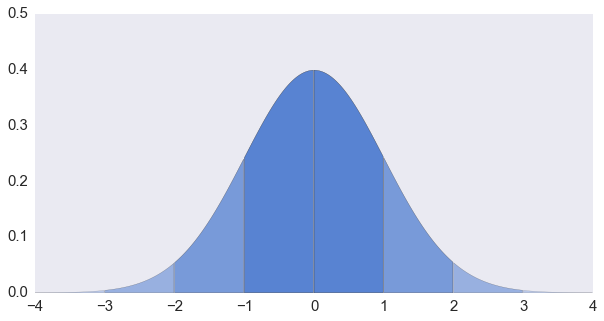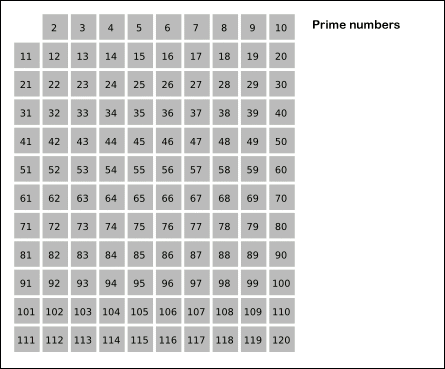正态分布随机数的生成 (2)

2015年11月16日 1 条评论

接受—拒绝法正态分布随机数的生成 (1)

2015年11月1日 没有评论

$$f(x \; | \; \mu, \sigma) = \frac{1}{\sigma\sqrt{2\pi} } \; e^{ -\frac{(x-\mu)^2}{2\sigma^2} }$$蓄水池抽样浅说 (3)

2015年10月23日 没有评论

加个权吧

$$P_i = \frac{w_i}{w_1 + w_2 + \cdots + w_n}$$

$$P_n(1) = \frac{w_n}{w_1 + w_2 + \cdots + w_n}$$

$$P_{n-1}(2) = \frac{w_{n-1}}{w_1 + w_2 + \cdots + w_{n-1}}$$

$$P(S) = \prod_{i=1}^n \frac{w_i}{w_1+w_2+\cdots w_i}$$

蓄水池抽样浅说 (2)

2015年10月22日 没有评论

跳跳跳！—— Algorithm X

• 确定该跳过多少个元素 $S(k, n)$
• 跳过 $S(k, n)$ 个元素
• 从前 $k$ 个元素中随机产生一个要替换的元素，用下一个元素替换

蓄水池抽样浅说 (1)

2015年10月21日 没有评论素数求和的动态规划方法

2015年9月29日 没有评论